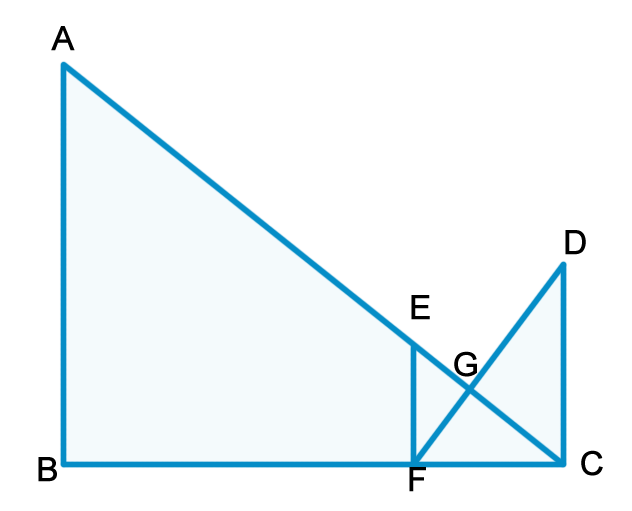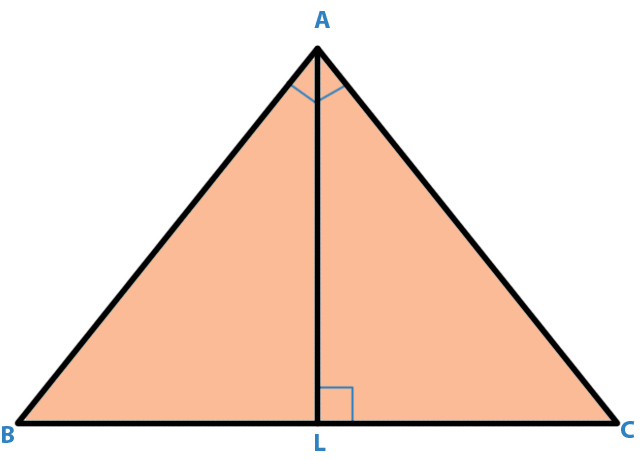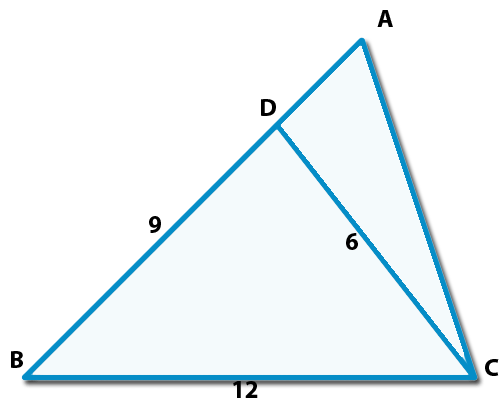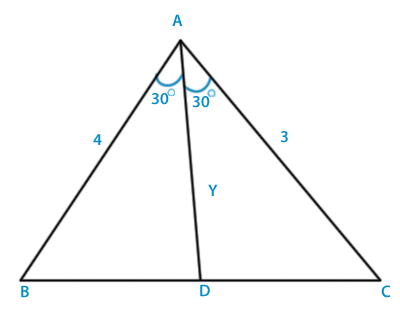# Triangle

## Triangles

 Question 1
In the adjoining figure AB, EF and CD are parallel lines. Given that GE = 5 cm, GC = 10 cm and DC = 18 cm, then EF is equal to:A 11 cm B 6 cm C 5 cm D 9 cm
Question 1 Explanation:
In $\Delta$ GEF and $\Delta$ GCD, we have

EFG = ∠GCD (Alternative angle)

EFG = ∠CGD(Vertically opposite angles)

$\Delta$ GEF ~ $\Delta$ GCD

Thus,

GE/CG = EF/CD

or, 5/10 = EF/18

or, EF = 9 cm.

 Question 2
A triangle cannot be drawn with the following three sides
 A 5m, 7m, 10m B 2m, 3m, 4m C 3m, 4m, 8m D 4m, 6m, 9m
Question 2 Explanation:
Addition any two side of a triangle is always greater then another side then only triangle formation is possible lets e.g a, b, c is a side of a triangle then a+b>c, b+c >a, c+a>b

So option b is not satisfying the condition 3 + 4 $\not\gt$ 8

 Question 3
The point of intersection of the altitudes of a triangle is called its:
 A Excentre B Orthocentre C Incentre D Centroid
Question 3 Explanation:
Orthocentre.

 Question 4
In a triangle ABC,∠ A = 900, AL is drawn perpendicular to BC, Then ∠ BAL is equal to:A ∠ALC B ∠BAC C ∠ACB D ∠B - ∠BAL
Question 4 Explanation:
∠ BAL + ∠ B + 900 = 1800

or, ∠ BAL + ∠ B = 900

or, ∠ BAL = 900 - ∠ B ----------- (1)

Now, in $\Delta$ ABC,

∠ ACB + ∠ B + ∠ A = 1800

∠ ACB = 900

-∠ B ----- (2)

From, (1) and (2),

∠ BAL = ∠ ACB.

 Question 5
In $\Delta$ PQR, PS is the bisector of ∠ P and PT $\perp$ OR, then ∠ TPS is equal to:
 A 900 + 1/2 ∠Q B 900 - 1/2 ∠R C 1/2 (∠ Q - ∠ R) D ∠Q + ∠ R
Question 5 Explanation:
∠1 + ∠2 = ∠3 [PS is bisector.] ------ (1)

∠Q = 900 - ∠1

∠R = 900 -∠2 - ∠3

So,

∠Q - ∠R = (900 - ∠1) - (900 - ∠2 - ∠3)

∠Q - ∠R = ∠2 + ∠3 - ∠1

∠Q - ∠ R = ∠2 + (∠1 + ∠2) -∠1[using equation 1]

∠Q - ∠R = 2 ∠2

1/2 * (∠Q - ∠R) = ∠TPS.

 Question 6
In $\Delta$ ABC, AD $\perp$ BC, then
 A AB2 - BD2 = AC2 - CD2 B AB2 - AC2 = BD2 + CD2 C AB2 + BD2 = AC2 - CD2 D AB2 - BD2 = AC2 + CD2
Question 6 Explanation:
In $\Delta$ ADC

AB2 = AD2 + BD2 ------- (1)

In Right angled $\Delta$ ACD,

AC2 = AD2 + BD2 ------- (2)

By (1) - (2)

AB2 - AC2 = BD2 - CD2

AB2 - BD2 = AC2 - CD2.

 Question 7
Consider the following statements :

I. Every equilateral triangle is necessarily an isosceles triangle.

II. Every right-angled triangle is necessarily an isosceles triangle.

III. A triangle in which one of the median is perpendicular to the side it meets, is necessarily an isosceles triangle.

The correct statements are:

 A I, II and III B I and III C II and III D I and II
Question 7 Explanation:
Every equilateral triangle is necessarily an isosceles triangle.

A triangle in which one of the median is perpendicular to the side it meets, is necessarily an isosceles triangle.

 Question 8
Consider the triangle shown in the figure where BC = 12 cm, Db = 9 cm, CD = 6 cm and What is the ratio of the perimeter of the triangle ADC to that of the triangle BDC ?A 5 : 9 B 8 : 9 C 6 :9 D 7 : 9
Question 8 Explanation:
Here, ∠ACB = c+180-(2c-b) = 180-(b+c)

So, We can say that $\Delta$ BCD and &delta ABC will be similar.

According to property of similarity,

AB/12 = 12/9

Hence,

AB = 16

AC/6 = 12/9

AC = 8

Hence, AD = 7 and AC = 8

Now,

Perimeter of Delta; ADC / Perimeter of &delta BDC,

= (6+7+8)/(9+6+12)

= 21/27 = 7/9.

 Question 9
In a triangle ABC, the internal bisector of the angle A meets BC at D. If AB = 4, AC = 3 and A = 600, then length of AD is :
 A 2 $\sqrt{3}$ B (6 $\sqrt{3}$) / 7 C (12 $\sqrt{3}$) / 7 D (15 $\sqrt{3}$) / 8
Question 9 Explanation:Let BC = x and Ad = y, then as per bisector theorem,

BD /DC = AB /AC = 4 /3

Hence, BD = 4x/7 and DC = 3x/7

Now, in $\Delta$ ABD using cosine rule,

cos 300 = [{42 +y2 - (16x2/49)} /2*3*y]

Or, 4 $\sqrt{3y}$ =[{16 +y2 - (16x2/49)}] --------- (i)

Similarly in $\Delta$ ADC,

cos 300 = [{32 +y2 - (9x2/49)} /2*3*y]

Or, 3 $\sqrt{3y}$ =[{9 +y2 - (9x2/49)}] --------- (ii)

From equation (i) and (ii), we get

y = 12 $\sqrt{3 }$/7.

 Question 10
In triangle PQR length of the side QR is less than twice the length of the side PQ by 2 cm. Length of the side PR exceeds the length of the side PQ by 10 cm. The perimeter is 40 cm. The length of the smallest side of the triangle PQR is :
 A 6 cm B 8 cm C 10 cm D 7 cm
Question 10 Explanation:
In $\Delta$ PQR,

QR +2 = 2PQ

QR = 2PQ - 2 ------- (2)

PR = PQ + 10 ----- (2)

Perimeter = 40 m

PQ + QR + Rp = 40

Putting the value of PQ and QR from equation (1) and (2),

Pq + 2PQ - 2 + PQ +10 = 40

4PQ = 32

PQ = 8 cm which is the smallest side of the triangle.

There are 10 questions to complete.